Next: The Vortex Core Up: Superconductivity Previous: BCS Theory

# Ginzburg-Landau Theory

The Ginzburg-Landau theory revolves around the concept of a complex order parameter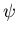, a pseudowavefunction describing the centre of mass motion of the Cooper pairs . The distribution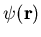is directly proportional to the superelectron density  ns(r) . This theory assumes local electrodynamics and is strictly valid only at temperatures near the transition temperature Tc . The order parameter must be small and vary slowly to derive the Ginzburg-Landau differential equations.

The Ginzburg-Landau differential equations proceed from minimising the Helmholtz free energy f of the superconducting state . The free energy f is expanded in powers of the order parameterand its spatial derivative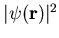, and the effect of a magnetic field on a particle of charge e* and mass m* is included. Minimising the resulting free energy expression with respect to the order parameterand the internal field  B(r)gives the Ginzburg-Landau equations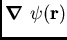where  A(r) is the vector potential associated with the internal field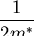. The quantities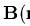and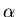are expansion coefficients which depend on the penetration depth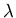and the thermodynamic critical field Hc. Relations (2.12) and (2.13) form coupled differential equations for the order parameterand the vector potential  A(r). The first equation resembles the Schrödinger equation for a free particle plus a nonlinear term. This nonlinear term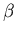encourages the order parameterto spread evenly throughout space. The second equation quantum mechanically describes a current of particles of charge e* and mass m*. Calculations based on the microscopic BCS theory show that the effective charge e* is twice the usual electronic charge e. Equation (2.12) also hints at another important length scale for superconductivity, the temperature dependent coherence length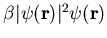.

The temperature dependent coherence length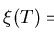characterizes the distance over which changes in the order parameteroccur . For this reason a finite coherence lengthimplies a gradual spatial evolution between superconducting and normal regions. Unlike the temperature independent BCS coherence length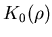, the Ginzburg-Landau coherence lengthgrows with temperature T in a manner similar to the penetration depth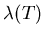.

The ratio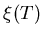of the penetration depth of a superconductor to its coherence length determines whether this material exhibits a vortex lattice. Figure 2.2 depicts the scenarios associated with the two extremes of the Ginzburg-Landau parameter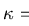.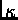In both cases the order parameter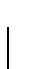rises from zero to its maximum value, and the internal field  B drops from its maximum to zero, across the border from normal to superconducting domains. In the situationa zone exists where the field  B has been substantially expelled and the order parameteris not yet maximal. Here the condensation energy is too small to completely compensate for the Gibbs free energy increase caused by the negative magnetisation. This leads to a positive surface energy in connection with the domain wall between the superconducting and the normal material. Conversely, in the case, a boundary region arises where the positive diamagnetic energy is not enough to counteract the Gibbs free energy reduction stemming from the growing number of superelectrons. The surface energy is now negative. Calculations reveal that the surface energy of a domain wall becomes zero for a Ginzburg-Landau parameter of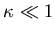. Type I labels superconductors with asmaller than this, while type II refers to those with a greater. In type II superconductors the negative surface energy generates a regular array of flux tubes, each associated with a single flux quantum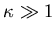. A flux line, also known as a vortex, is quasinormal at its centre, where the order parameter, the supercurrent density  J(r)and the BCS energy gap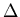all vanish. The detailed structure of a vortex forms the subject of the next section.Next: The Vortex Core Up: Superconductivity Previous: BCS Theory
Jess H. Brewer
2001-10-31